# Class 8 RD Sharma Solutions – Chapter 17 Understanding Shapes Special Types Of Quadrilaterals – Exercise 17.2 | Set 2

• Last Updated : 21 Feb, 2021

### Question 9. One side of a rhombus is of length 4 cm and the length of an altitude is 3.2 cm. Draw the rhombus.

Solution: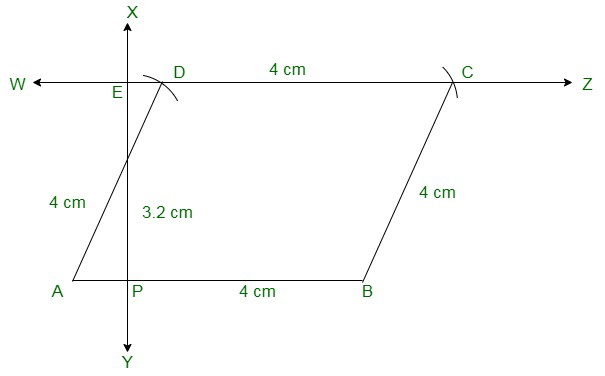Attention reader! All those who say programming isn't for kids, just haven't met the right mentors yet. Join the  Demo Class for First Step to Coding Coursespecifically designed for students of class 8 to 12.

The students will get to learn more about the world of programming in these free classes which will definitely help them in making a wise career choice in the future.

Steps to construct a rhombus:

(i) Draw a line segment of 4 cm

(ii) From point A draw a perpendicular line bisecting the length of 3.2 cm to get point E.

(iii) From point E draw a line parallel to AB.

(iv) From points A and B cut two arcs of length 4 cm on the drawn parallel line to get points D and C.

(v) Join the line segments AD, BC and CD to get rhombus ABCD.

### Question 10. Draw a rhombus ABCD, if AB = 6 cm and AC = 5 cm.

Solution: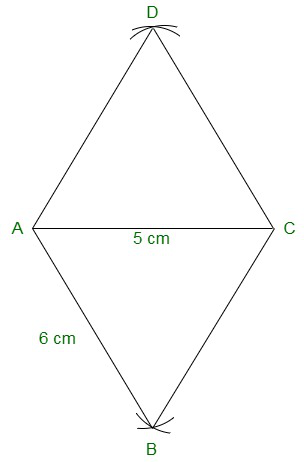Steps of construction:

(i) Draw a line segment AB of length 6 cm.

(ii) From point ‘A’ cut an arc of length 5 cm and from point B cut an arc of length 6 cm intersecting at ‘C’.

(iii) Join the line segments AC and BC.

(iv) From point A cut an arc of length 6 cm and from point C cut an arc of 6cm, so that both the arcs intersect at point D.

(v) Join the remaining line segments AD and DC to get rhombus ABCD.

### Question 11. ABCD is a rhombus and its diagonals intersect at O.

(i) is ΔBOC ≅ ΔDOC? State the congruence condition used?

(ii) Also state, if ∠BCO = ∠DCO.

Solution: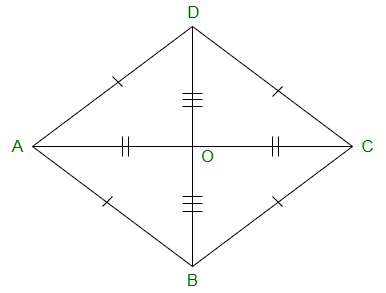(i) Yes,

In ΔBOC and ΔDOC

Since, in a rhombus diagonals bisect each other, we have,

BO = DO

CO = CO Common

BC = CD [All sides of a rhombus are equal]

Now,

By using SSS Congruency, ΔBOC≅ΔDOC

(ii) Yes.

Since by,

∠BCO = ∠DCO, by corresponding parts of congruent triangles.

### Question 12. Show that each diagonal of a rhombus bisects the angle through which it passes.

Solution: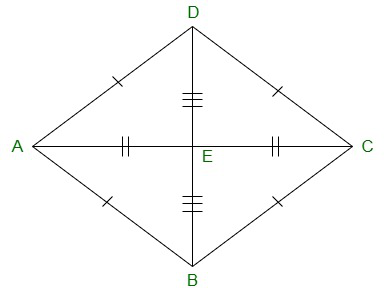(i) In ΔBOC and ΔDOC

BO = DO [In a rhombus diagonals bisect each other]

CO = CO Common

BC = CD [All sides of a rhombus are equal]

By using SSS Congruency, ΔBOC≅ΔDOC

∠BCO = ∠DCO, by corresponding parts of congruent triangles

Therefore,

Each diagonal of a rhombus bisect the angle through which it passes.

### Question 13. ABCD is a rhombus whose diagonals intersect at O. If AB=10 cm, diagonal BD = 16 cm, find the length of diagonal AC.

Solution: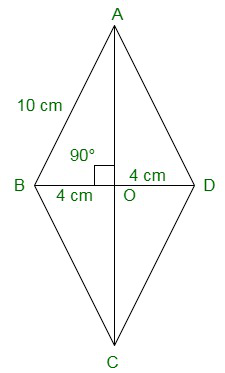In a rhombus diagonals bisect each other at right angle.

In ΔAOB

BO = BD/2 = 16/2 = 8cm

AB2 = AO2 + BO2 (Pythagoras theorem)

102 = AO2 + 82

100-64 = AO2

AO2 = 36

AO = √36 = 6cm

Hence, Length of the diagonal AC is 6 × 2 = 12cm.

### Question 14. The diagonal of a quadrilateral are of lengths 6 cm and 8 cm. If the diagonals bisect each other at right angles, what is the length of each side of the quadrilateral?

Solution: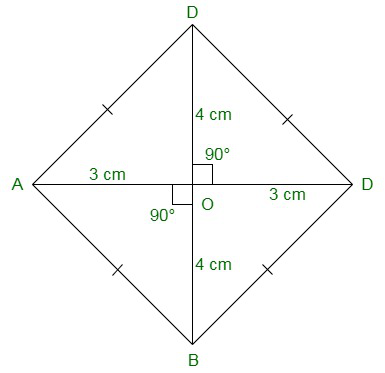In a rhombus diagonals bisect each other at right angle.

Considering ΔAOB

BO = BD/2 = 6/2 = 3cm

AO = AC/2 = 8/2 = 4cm

Now,

AB2 = AO2 + BO2 (Pythagoras theorem)

AB2 = 42 + 32

AB2 = 16 + 9

AB2 = 25

AB = √25 = 5cm

Hence, Length of each side of the quadrilateral ABCD is 5cm.

My Personal Notes arrow_drop_up